Courses

# Test: Stress-Strain Relations & Elastic Constants - 1

## 10 Questions MCQ Test Topicwise Question Bank for Mechanical Engineering | Test: Stress-Strain Relations & Elastic Constants - 1

Description
This mock test of Test: Stress-Strain Relations & Elastic Constants - 1 for Mechanical Engineering helps you for every Mechanical Engineering entrance exam. This contains 10 Multiple Choice Questions for Mechanical Engineering Test: Stress-Strain Relations & Elastic Constants - 1 (mcq) to study with solutions a complete question bank. The solved questions answers in this Test: Stress-Strain Relations & Elastic Constants - 1 quiz give you a good mix of easy questions and tough questions. Mechanical Engineering students definitely take this Test: Stress-Strain Relations & Elastic Constants - 1 exercise for a better result in the exam. You can find other Test: Stress-Strain Relations & Elastic Constants - 1 extra questions, long questions & short questions for Mechanical Engineering on EduRev as well by searching above.
QUESTION: 1

### The independent elastic constants for homogeneous and isotropic material are

Solution:

Only two independent elastic constant are required to express the stress-strain relation for a linearly elastic, isotropic material. We can find third elastic constant and Poisson’s ratio for the material.

QUESTION: 2

Solution:
QUESTION: 3

### If the Young’s modulus E is equal to bulk modulus K, then the value of Poisson’s ratio is

Solution:

E = 3 K ( 1 - 2 v)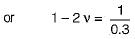(∴ E = K)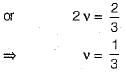QUESTION: 4

A 50 cm long x 20 cm diameter cylinder of brass was subjected to a tensile load of 0.1 MN. The resulting increase in length and decrease in diameter were noted 1 mm and 0.1 mm respectively. Then the brass has a Poisson’s ratio equal to

Solution: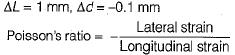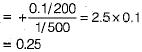QUESTION: 5

Consider a bar of length L, breadth B and thickness t subjeced to an axial pull or tension P. The resulting volumetric strain will be equal to

Solution: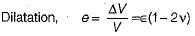where v is the Poisson’s ratio and ∈ is the longitudinal strain.

QUESTION: 6

The Young's modulus and the Poisson’s ratio for a certain material are stated to be 1000 GPa and 0.25 respectively. The bulk modulus of the material will be about

Solution:

E = 3 K ( 1 - 2 v )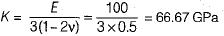QUESTION: 7

If E, G and K denote the Young’s modulus, modulus of rigidity and bulk modulus for an elastic material, then which one of the following can be possibly true?

Solution:

Most of the elastic material,
v = 0.25 to 0.33
so, for v = 0.25, E = K x 3(1 - 0.5 ) = 1.5 K
and v = 0.33,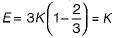(Possible relation)

QUESTION: 8

A spherical ball of 0.5 x 103 mm3 volume is subjected to a volumetric stress of 0.5 MPa. If bulk modulus of the bail material is 16 GPa, the change in volume of the ball would be approximately equal to

Solution:

Bulk modulus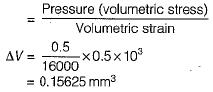QUESTION: 9

For an isotropic, homogeneous and elastic material obeying Hooke’s law, number of independent elastic constants are

Solution:
QUESTION: 10

The number of elastic constants for a completely anisotropic elastic material are

Solution: